• 基于matlab的音频信号处理毕业设计(含源文件
千次阅读
2021-09-08 11:55:28

基于MATLAB的语音信号处理GUI设计

## 一、课题研究的意义

本课题旨在对音频文件的调制和滤波。音频信号处理是研究用数字信号处理技术和语音学知识对语音信号进行处理的新兴的学科，是目前发展最为迅速的信息科学研究领域的核心技术之一。通过声音传递信息是人类最重要、最有效、最常用和最方便的交换信息形式。同时，声音也是人与机器之间进行通信的重要工具，它是一种理想的人机通信方式，因而可为信息处理系统建立良好的人机交互环境，进一步推动计算机和其他智能机器的应用，提高社会的信息化程度。

语音信号是基于时间轴上的一维数字信号，在这里主要是对语音信号进行频域上的分析。在信号分析中，频域往往包含了更多的信息。对于频域来说，大概有8种波形可以让我们分析：矩形方波，锯齿波，梯形波，临界阻尼指数脉冲波形，三角波，余旋波，余旋平方波，高斯波。对于各种波形，我们都可以用一种方法来分析，就是傅立叶变换：将时域的波形转化到频域来分析。

语音信号处理是一门新兴的学科，同时又是综合性的多学科领域和涉及面很广的交叉学科。虽然从事这一领域研究的人员主要来自信号与信息处理及计算机应用等学科，但是它与语音学、语言学、声学、认知科学、生理学、心理学等许多学科也有非常密切的联系。

## 二、课题发展历程和现状

在60年代，计算机的广泛应用推动了语音识别技术的发展，出现了多种语音信号分析方法，提出了动态规划(DP)和线性预测分析(LP)技术。其中后者较好地解决了语音信号产生模型的问题，对语音识别的发展产生了深远影响。 70年代，语音识别理论取得了突破。LP技术得到进一步发展，动态时间归正技术(DTW)基本成熟，特别是提出了矢量量化(VQ)和隐马尔可夫模型(HMM)理论。在实践上，实现了基于线性预测倒谱和DTW技术的特定人孤立语音识别系统。 80年代，HMM模型和人工神经元网络(ANN)成功应用于实践，并于1988年由CMU研制出了非特定人、大词汇量、连续语音识别系统—SPHINX。它可以理解由1000个单词构成的4200个句子，被认为是语音识别历史上的一个里程碑。HMM模型的广泛应用应归功于AT&T Bell实验室Rabiner等科学家的努力，他们把原本晦涩难懂的HMM纯数学模型工程化，从而为更多研究者了解和认识。ANN和HMM模型建立的语音识别系统，性能相当。 进入90年代，随着多媒体时代的来临，迫切要求语音识别系统从实验室走向实用。许多著名的大公司如IBM、苹果、AT&T和NTT都对语音识别系统的实用化研究投以巨资。

语音识别技术有一个很好的评估机制，那就是识别的准确率，而这项指标在20世纪90年代中后期实验室研究中得到了不断的提高。比较有代表性的系统有：IBM公司推出的Via Voice和Dragon System公司的Naturally Speaking Nuance公司的Nuance Voice Platform语音平台，Microsoft的Whisper Sun的Voice Tone等。语音识别也得到了更多的商用，其中SpeechWorks6是世界领先的电话自动语音识别系统解决方案提供者—SpeechWorks公司的代表产品。利用该产品，用户可以通过电话用自然语言与系统进行交互，进行旅游预约、股票交易、银行服务、订票服务、宾馆服务和寻呼服务等，无需服务人员的介入。目前市场上出现了语音识别电话、语音识别记事本等产品，如美国VPTC公司的VoiceOrganizer和法国的Parrot等。

在我国，语音技术的研究起步较晚，70年代末才开始了语音技术的研究，但在很长一段时间内，都处于缓慢发展的阶段，直到80年代后期，随着计算机应用技术在我国的逐渐普及和数字信号处理技术的进一步发展，国内许多单位纷纷投入到这项研究工作中去，其中有中科院声学所，自动化所，清华大学，四川大学和西北工业大学等科研机构和高等院校，大多数研究者致力于语音识别的基础理论研究工作、模型及算法的研究和改进。但由于起步晚、基础薄弱、计算机水平不发达，导致在整个80年代，我国在语音识别研究方面并没有形成自己的特色，更没有取得显著的成果和开发出大型性能优良的实验系统。

## 三、实现功能

在本课题中，所要研究的内容首先是语音的录入部分和对录入的音频数据进行频谱的分析并画出其图像。其次是设计滤波器并对加入噪声后的音频文件进行滤波并考察其使用情况以此来检验滤波器的设计是否正确。

本课题研究内容包括：

2. 图形用户界面GUI的设计：包含设置界面及按钮并对各按钮功能能进行编程。需要实现按键执行信号播放，加噪声和滤波的功能。
3. 利用傅里叶变换求信号的频域并作图：使用MATLAB中的傅里叶变换命令对已经采集到的音频信号做傅里叶变换，变换完成后画出其在频域上的波形并对原时域上的波形进行对比。
4. 对采样后的信号进行加噪声处理：使用matlab中的相关命令。WGN， AWGN。WGN用于产生高斯白噪声，AWGN则用于在某一信号中加入高斯白噪声。也可直接用randn函数产生高斯分布序列。
5. 设计数字滤波器：设计一个数字滤波器时，根据指标先写出模拟滤波器的公式，再通过一定的变换，将模拟滤波器的公式转换成数字滤波器的公式。

## 四、 算法流程图

开始工作

对音频信号采集

音频信号的处理

验证滤波效果

添加噪声

对原信号进行傅里叶变换

使用滤波器对信号滤波

画图观察采集信号的时域波形

画图观察变换后信号的频域波形

使用不同种类的滤波器，观察区别

## 5.1 音频的采集与作图

以下展示的程序为语音信号在MATLAB中操作的表现，它实现了语音的读入打开，并利用FFT快速傅里叶变换绘出了语音信号的波形图频谱图。

clear all;
music=input('输入文件名：','s')
sound(y,fs,nbits);                   %音频信号的播放
n=length(y);                         %设定长度
Y=fft(y,n);                          %快速傅里叶变换
figure;                              %控窗
subplot(2,1,1);
plot(y,’r’);
title('波形图','fontweight','blod');
grid;                                %网格
subplot(2,1,2);
plot(abs(Y),’b’);                  %取绝对值
title('频谱图','fontweight','blod');
grid;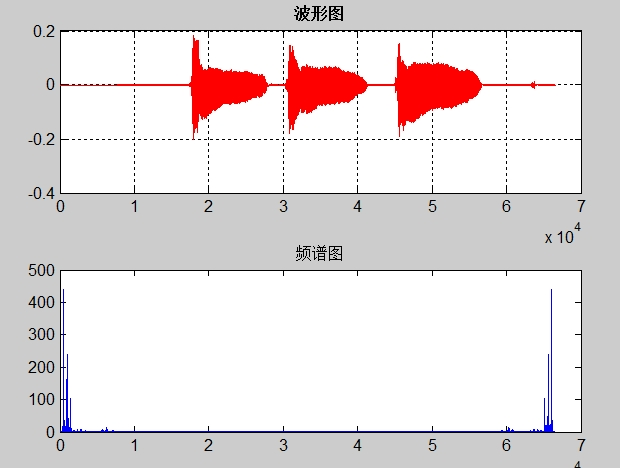如图所示，展示的是一段语音信号的时域和频域波形

## 5.2对采集信号进行加噪声处理。

MATLAB中产生高斯白噪声的两个函数

MATLAB中产生高斯白噪声非常方便，可以直接应用两个函数，一个是WGN，另一个是AWGN。WGN用于产生高斯白噪声，AWGN则用于在某一信号中加入高斯白噪声。

在设计中使用的是直接产生高斯噪声的RANDN函数，实验采用的是MATLAB中的随机函数rand产生噪声加入到语音信号中，通过对噪声信号的加入以此来实现模仿语音信号被污染，并对添加噪声后的文件进行频谱分析。

matlab函数randn：产生正态分布的随机数或矩阵的函数

randn

产生均值为0，方差 σ^2 = 1，标准差σ = 1的正态分布的随机数或矩阵的函数。

用法：

Y = randn(n)

返回一个n*n的随机项的矩阵。如果n不是个数量，将返回错误信息。

Y = randn(m,n) 或 Y = randn([m n])

返回一个m*n的随机项矩阵。

Y = randn(m,n,p,...) 或 Y = randn([m n p...])

产生随机数组。

Y = randn(size(A))

返回一个和A有同样维数大小的随机数组。

在本次实验过程中，我们选用Randn（m,n）函数。对语音信号添加噪声及其频谱分析的主要程序如下：

clear all;
music=input('输入文件名：','s')
n = length (y)
Noise=0.3*randn(n,2);   %编辑噪声
s=y+Noise;
Y=fft(y,n);
sound(s);
S=fft(s);
figure;
subplot(2,2,1);
plot(y,'r');
title('时域波形图','fontweight','bold');
grid;
subplot(2,2,2);
plot(abs(Y),'r');
title('频域波形图','fontweight','blod');
subplot(2,2,3);
plot(s);
title('加噪时域波形图','fontweight','bold');
grid;
subplot(2,2,4);
plot(abs(S));
title('加噪频域波形图','fontweight','bold');
grid;
;


程序运行结果如下：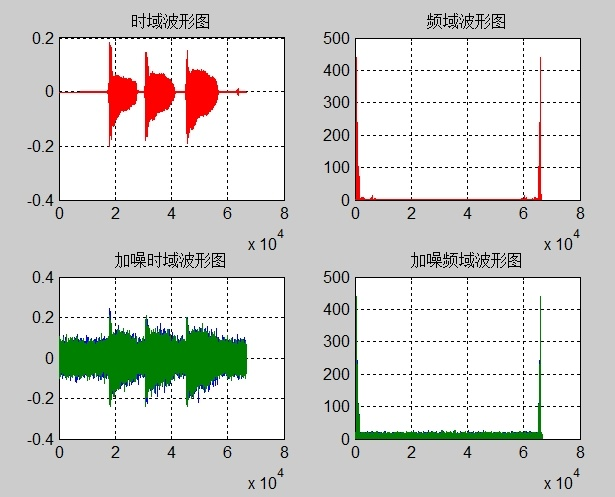加噪后信号波形图

## 5.3 扩展内容：量标准化

运行程序如下：

clear all;
music=input('输入文件名：','s')
ym=max(max(max(y)),max(abs(min(y))));
x=y/ym;
figure;
subplot(2,1,1);
plot(y,’r’);
title('原图','fontweight','blod');
grid;                                %网格
subplot(2,1,2);
plot(x,’b’);
title('改图','fontweight','blod');
grid;
sound(x)
wavwrite(x,fs,bits,'2.wav');


程序内容为：对原语音信号处理保存后播放并画出时域波形图，有音量增大的效果。但于实际测试播放时声音会有一定程度的失真。

程序运行结果：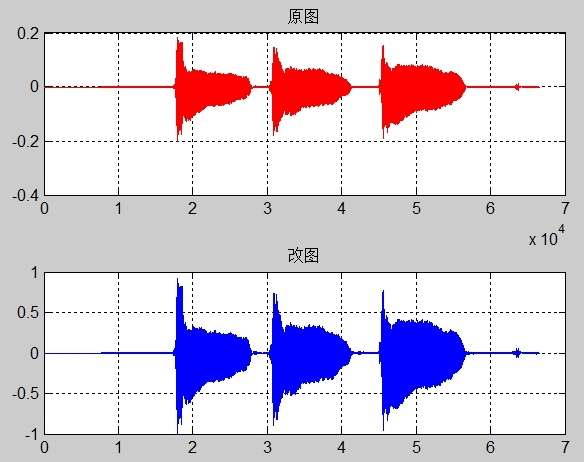如图，运行后相较于原波形图有了一定程度的幅度提高。

## 5.4扩展内容2：信号的重采样

在语音信号的分析与处理过程中往往会对原语音信号进行重采样处理。经过重采样后能够减少程序的运行负担。在低配置的电脑系统中较为重要。

同时，重采样还能够带来降频和倍频的功能。在平时的信号处理中这种变换调整也经常要用到。所以在设计中也添加了这种功能，通过相关的处理可以使语音信号的声音特征产生一定的变化。在本次实验中，常通过重采样功能对声音信号进行调制。故在此进行部分说明。在本次软件使用时重采样功能多用于对原声音文件进行降频。

以下为本次实验中使用的重采样功能的部分代码：

fre=get(handles.popagainhz,'string');
popup_sel_index = get(handles.popagainhz, 'Value');
switch popup_sel_index
case 1
fre=1000;
case 2
fre=2000;
case 3
fre=4000;
end
figure(4);
subplot(2,2,1);
plot(ft);
title('原始信号波形');xlabel('时间');ylabel('幅度');
subplot(2,2,2);
Y=fft(ft);
plot(abs(Y));
title('原始信号频谱');xlabel('频率');ylabel('幅度');
%重采样
y2=resample(ft,1,Fs/fre);
sound(y2,fre);
subplot(2,2,3);
plot(y2);
title('重采样后信号波形');xlabel('时间');ylabel('幅度');
subplot(2,2,4);
Y2=fft(y2);
plot(abs(Y2));
title('重采样后信号频谱)');xlabel('时间');ylabel('幅度');


## 六、参考文献

 郑春佳、陈仅星、陈金西，【数字信号处理-基于数值计算】西安电子科技大学出版社.

 刘卫国，【matlab程序设计与应用】高等教育出版社.

 胡航，【语音信号处理】哈尔滨工业大学出版社.

 刘幺和、宋庭新，【语音识别与控制技术】，科学出版社.

 苏金明、张莲花、刘波，【MATLAB工具箱应用】，电子工业出版社.

 赵新敏，（语音信号的识别技术），学位论文，天津大学.

 网友，（基于MATLAB的有噪声引号处理），学位论文，南京大学

 王京辉，(语音信号处理技术研究) 论文，山东大学.

 赵力，语音信号处理，机械工业出版社.

 陈杰，【Matlab宝典】电子工业出版社.

 (美) Sanjit K. Mitra著；孙洪, 余翔宇等译.【数字信号处理实验指导书】

 杨述斌，李永全，【数字信号处理实践教程】华中科技大学出版社

 Gersho A,Gr R M.Vector quantization and signalCompression,Boston,Kluwer Academic Publishers.机器学习 人工智能 大数据 编程语言
更多相关内容
• 声音信号进行处理的matlab仿真，可以用于水声通信领域。Matlab
• 信号系统：简单的声音信号处理实验报告-郑州轻工业学院 Matlab音频信号处理实验报告 Matlab 音频信号处理实验
• 数字信号处理，音频信号处理，A计权，三分之一倍频程等
• 基于matlab的一款声音处理软件，其中包括声音提取（现场录入或者文件导入），声音的FFT频谱分析，相位分析以及滤波处理，通过三种滤波方式对声音进行去噪以及具有50%以上精度的声纹识别系统。
• matlab实现声音信号的采集、加噪、低通、高通、带通滤波，回放声音信号
• MATLAB声音信号处理中的技术难点分析.pdf
• 课程设计任务书 学生姓名 XXX 学生学号 XXXXXXXXXXXXXXX 学生专业 XXXXXXXXXXXXXXX 学生班级 XXXXXXXXXXX 指导教师 XXXXXXXXXXX ...MATLAB的声音信号处理 设计目的 1巩固所学的数字信号处理理论知识 2理解信号的采集处
• 基于labview的简单声音信号处理。将声音信号进行模拟，将文件保存到文档中
• matlab实现声音信号处理的指导文档，包括声音采集、加噪、滤波的过程，描述详细。
• soundprocess_A计权_声音信号处理.zip
• 完整代码和详细报告，可直接运行，MATLAB创建GUI界面，实现数字信号处理、数字图像处理，数字巴特沃斯滤波器，切比雪夫1型滤波器，FIR滤波器
• soundprocess_A计权_声音信号处理_源码.zip
• 郑州轻工业大学实验五简单的声音信号处理报告-信号系统报告
• 实验一 基于MATLAB的语音信号时域特征分析 实验二 基于MATLAB分析语音信号频域特征 实验三 基于MATLAB的LPC分析。
• 语音信号处理实验素材 wav语音文件，可于MATLAB 语音信号处理实验
• ## 基于matlab的语音信号处理

万次阅读 多人点赞 2021-08-31 11:57:57
摘要利用所学习的数字信号处理知识，设计了一个有趣的音效处理系统，首先设计了几种不同的滤波器对声音进行滤波处理，分析了时域和频域的变化，比较了经过滤波处理后的声音与原来的声音有何变化。同时设计实现了语音...

摘要

利用所学习的数字信号处理知识，设计了一个有趣的音效处理系统，首先设计了几种不同的滤波器对声音进行滤波处理，分析了时域和频域的变化，比较了经过滤波处理后的声音与原来的声音有何变化。同时设计实现了语音的倒放，变速播放，回响，音调转换等处理效果，其中音调转换部分使用了重新采样改变基频，再进行时长规整的算法。

基于MATLAB的语音信号处理

语音信号的采集

d.wav 真心英雄（周华健）（男声）

man.wav我的歌声里（自己录制）（男声）

girl.wav看的最远的地方（张韶涵）（女声）

语音信号的频谱分析

使用matlab画出语音信号的时域波形;然后对语音号进行快速傅里叶变换,得到信号的频谱特性，画出频谱图，分析频率成分。这里我们分析的是d.wav，通过分析知道频率分布在0—10KHz，主要分布在低频。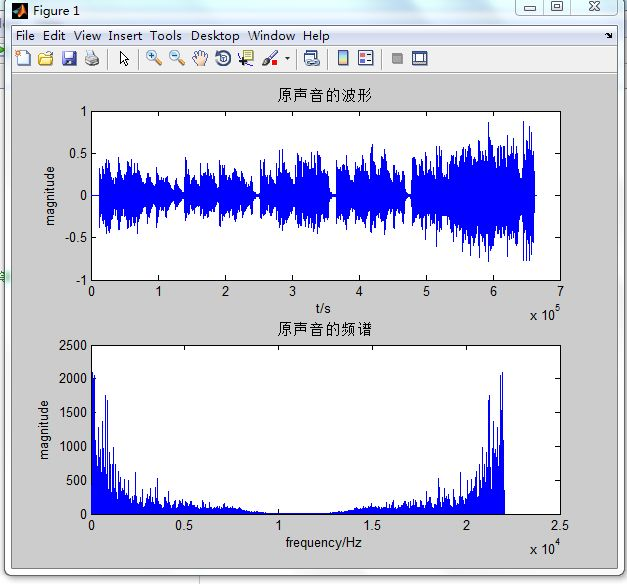数字滤波器设计

这里我们设计了4种滤波器对语音进行处理，分别为椭圆低通滤波，椭圆高通滤波，等波纹逼近法FIR带通滤波器，双线性变换法切比雪夫数字高通滤波器，绘制出相应的幅度、相位谱图，滤波后的波形、频谱图。

各滤波器的设计如下：

椭圆低通滤波器：fb＝1 200 Hz,fc＝1 400 Hz,As＝100 dB,Ap＝1 dB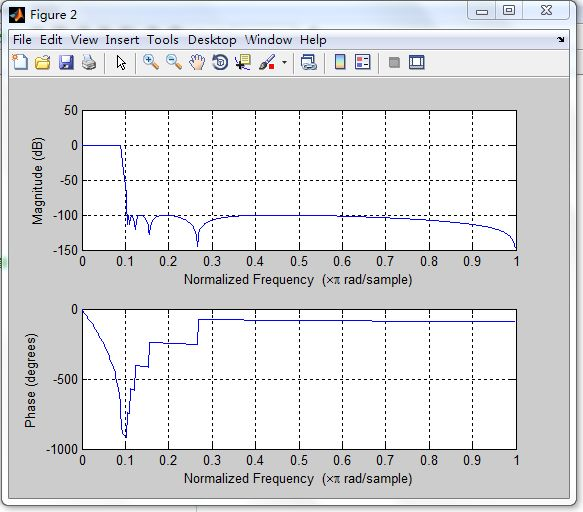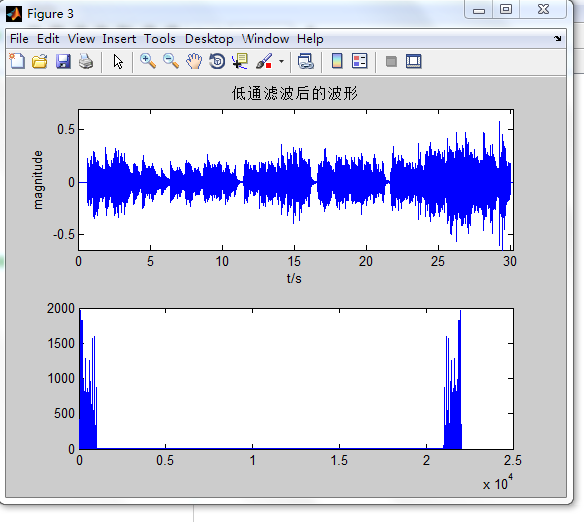椭圆高通滤波器：fc＝4 800 Hz,fb＝5 000 Hz As＝100 dB,Ap＝1 dB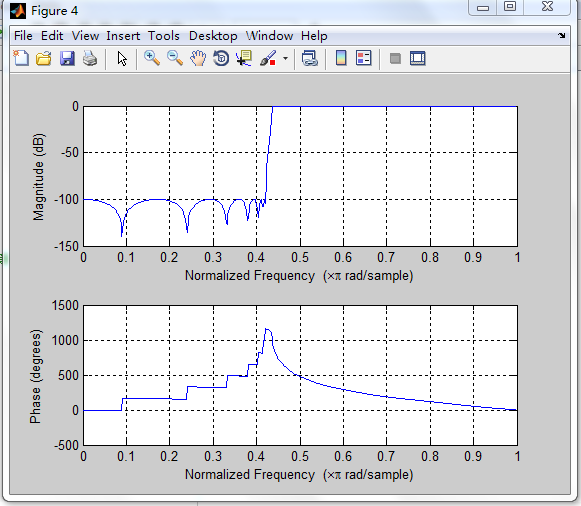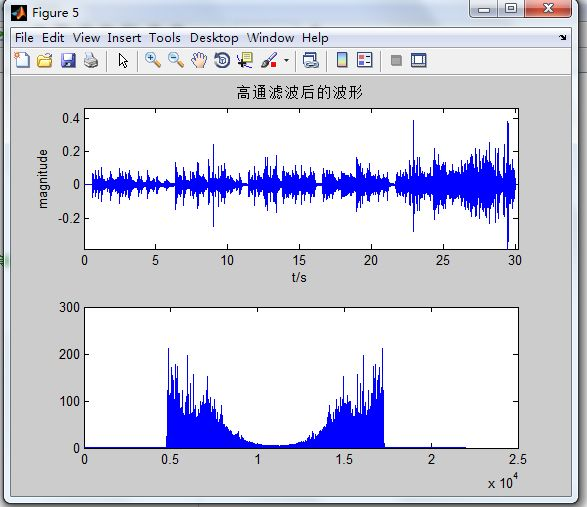等波纹逼近法设计FIR带通滤波器

fb1＝1200Hz,fb2＝3000Hz,fc1＝1000Hz,fc2＝3000Hz,As＝100dB,Ap＝1dB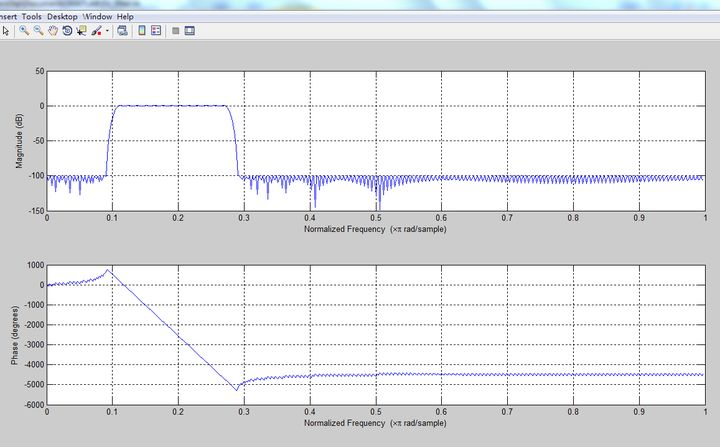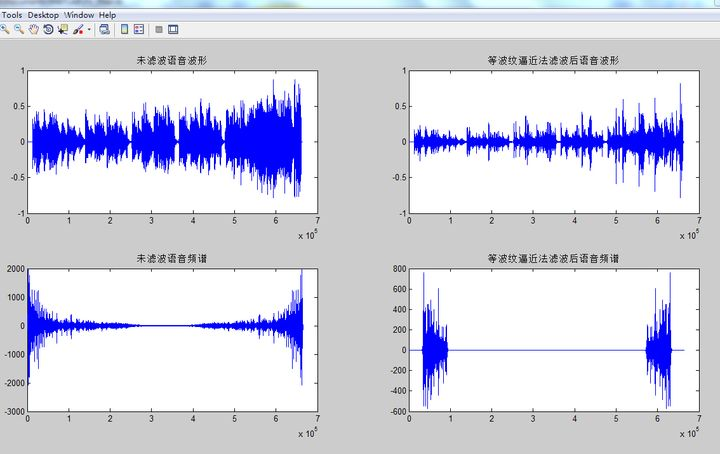双线性变换法切比雪夫数字高通滤波器

fc＝4 800 Hz,fb＝5 000 Hz As＝100 dB,Ap＝1 dB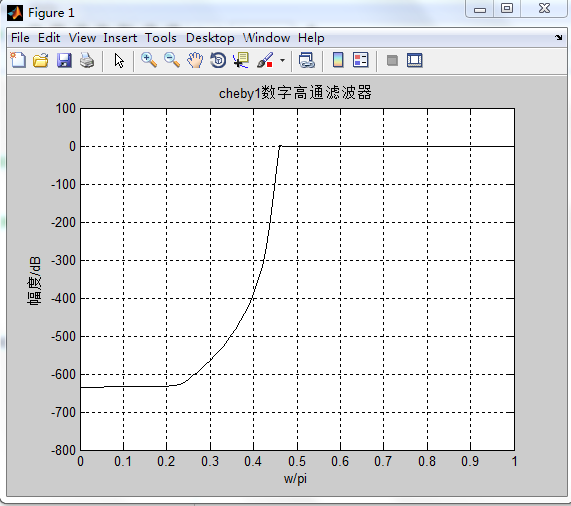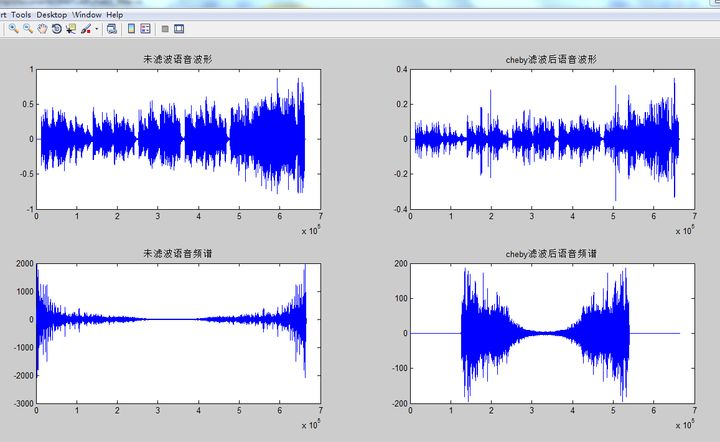滤波处理后，我们用函数sound()可以对声音进行回放,调用格式：sound(x,fs,bits);感觉滤波前后的声音。语音的低频部分沉稳，空间感较强;中频部分音质一般;高频部分音质非常尖锐，略微有尖音。此外中高频的幅度都不大，回放时音量较低。

语音变速播放

改变语音的播放速度也就是改变采样间隔（即改变了采样频率），但是这个频实现率依然要在2f(Nyquist rate)之上，否则就会产生失真。

%变速-慢放%

w=0.9;

M=w*fs; %w>1为快放，w<1为慢放

sound(x,M,nbits);

语音倒放

使用flipud()倒置语音矩阵，逆序输出音频即可。

回响效果

回声在时域上幅值减小了，频域上的特征不变，只需要把原信号添加一个延时（delay）和对时域的幅度添加一个参数.然后和原信号叠加即可获得回响效果。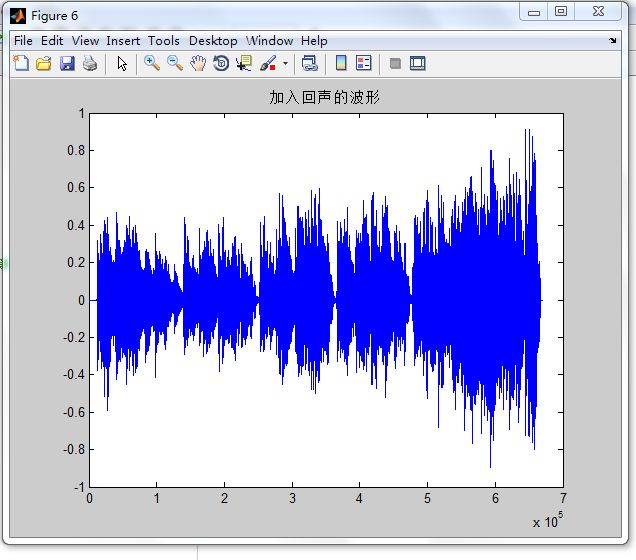实现男女声转换音效效果

我们使用另外写的voice(x,f)函数实现音调转换，x为需要转换的声音，通过抽取插值更改采样率来改变基频，当f>1时音调降低;f<1音调升高。然后再进行时长整合使语音文件恢复原来的时长。时长整合使用重叠叠加算法来实现。经过我们试听，转换效果还是很好的。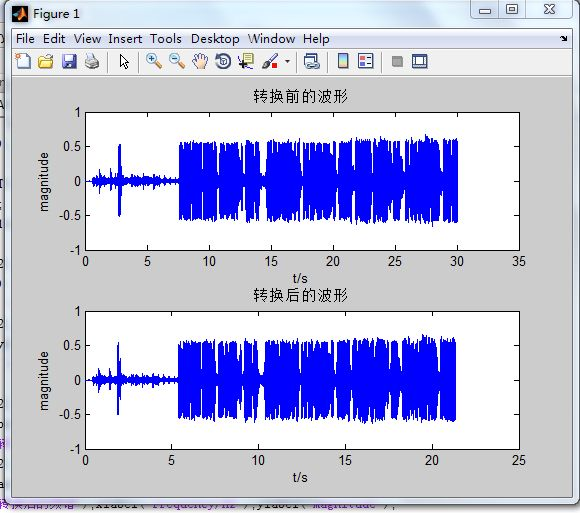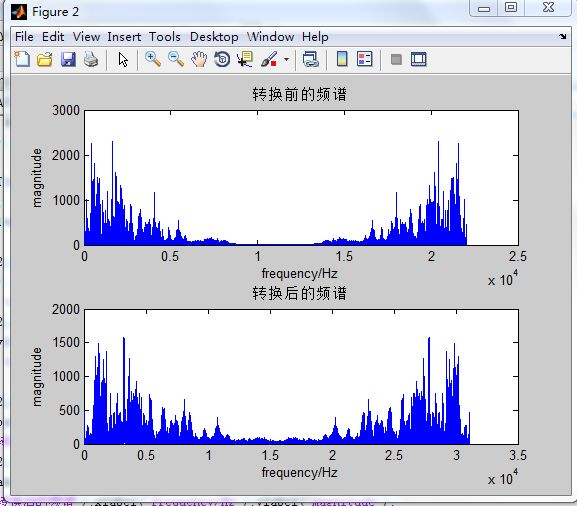总结体会

通过对声音信号的滤波处理，比较其前后变化，感受到了滤波器在声音信号处理当中的作用，同时在实践中掌握了滤波器的基本设计方法，加深了对各种类型的数字滤波器特性的理解。我们通过对声音的各种变换，产生了多种不同的音效，也体会到了语音处理的魅力。

代码附录

%读取声音信号%

x=y(:,1); %读入的y矩阵有两列,取第1列

N=length(x);

n=0:N-1;

X= fft(x); %傅里叶变换

Fs=2*fs; %2倍频

T=1/Fs;

f=n/N*Fs;

figure;

subplot(2,1,1);

plot(n,x); %声音的时域波形

title('原声音的波形');

xlabel('t/s');

ylabel('magnitude');

subplot(2,1,2);

plot(f,abs(X)); %声音的频谱

title('原声音的频谱');

xlabel('frequency/Hz');

ylabel('magnitude');

% 滤波器设计%

% 椭圆低通滤波器%

fp1=1200;fs1=1400; %低通滤波器通带截止频率1200Hz和阻带截止频率1400Hz

wp1=2*fp1/Fs; ws1=2*fs1/Fs;rp=1;as=100;

[N1,wp1]=ellipord(wp1,ws1,rp,as); %计算椭圆低通模拟滤波器的阶数和通带边界频率

[B,A]=ellip(N1,rp,as,wp1); %计算低通滤波器模拟滤波器系统函数系数

y1=filter(B,A,x); %滤波器软件实现

% 低通滤波器绘图部分%

figure;

freqz(B,A);

figure;

subplot(2,1,1);

t=n*T;

plot(t,y1);

xlabel('t/s');ylabel('magnitude');title('低通滤波后的波形');

axis([0,t(end),min(y1),1.2*max(y1)])%坐标范围

subplot(2,1,2);

plot(f,abs(fft(y1)));

%椭圆高通滤波器%

fp2=4800;fs2=5000; %高通滤波器通带截止频率5000Hz和阻带截止频率4800Hz

% 高通滤波器绘图部分%

figure;

freqz(B2,A2);

figure;

subplot(2,1,1);

t=n*T;

plot(t,y2);

xlabel('t/s');ylabel('magnitude');title('高通滤波后的波形');

axis([0,t(end),min(y2),1.2*max(y2)])

subplot(2,1,2);

plot(f,abs(fft(y2)));

%等波纹逼近法设计ＦＩＲ带通滤波器及滤波

y=I(:,1);

fp1=1200;fp2=3000;fc1=1000;fc2=3200;FS=2*fs;rp=1;rs=100;

f=[fc1,fp1,fp2,fc2];

m=[0,1,0];

dat1=(10^(rp/20)-1)/(10^(rp/20)+1);dat2=10^(-rs/20);

rip=[dat2,dat1,dat2];

[M,fo,mo,w]=remezord(f,m,rip,FS);

M=M+1;

hn=remez(M,fo,mo,w);

figure(1);

freqz(hn);

Y=fft(y);

y1=fftfilt(hn,y); %用remez设计的滤波器进行滤波

figure(2);

subplot(221);plot(y);title('未滤波语音波形');

subplot(222);plot(y1);title('等波纹逼近法滤波后语音波形');

subplot(223);plot(n,Y);title('未滤波语音频谱');

subplot(224);plot(n,Y1);title('等波纹逼近法滤波后语音频谱');

sound(y1,fs,nbits); %滤波后语音回放

%cheby1设计模拟高通滤波器再经双线性变换法设计成数字高通滤波器

fp=5000;fc=4800;rp=1;rs=100;FS=2*fs;

wpz=2*pi*fp/FS;wsz=2*pi*fc/FS;

wp=2*tan(wpz/2)*FS;ws=2*tan(wsz/2)*FS; %预畸校正转换指标

[N,wpo]=cheb1ord(wp,ws,rp,rs,'s');

[BH,AH]=cheby1(N,rp,wpo,'high','s');

[Bz,Az]=bilinear(BH,AH,FS);

w=0:0.01*pi:pi;

[h,w]=freqz(Bz,Az,w);

plot(w/pi,20*log(abs(h)),'k');axis([0,1,-800,100]);

xlabel('w/pi');ylabel('幅度/dB');grid;

title('cheby1数字高通滤波器');

Y=fft(y);

figure(2);

subplot(221);plot(y);title('未滤波语音波形');

subplot(222);plot(y1);title('cheby滤波后语音波形');

subplot(223);plot(n,Y);title('未滤波语音频谱');

subplot(224);plot(n,Y1);title('cheby滤波后语音频谱');

sound(y1,fs,nbits); %滤波后语音回放

%播放声音%

sound(x,fs,nbits); %原声

sound(5*y1,fs,nbits); %低通

sound(5*y2,fs,nbits); %高通

%变速-慢放%

w=0.9;

M=w*fs; %w>1为快放，w<1为慢放

sound(x,M,nbits);

%语音倒放%

y0=flipud(x);

sound(y0);

%回声%

z=[zeros(5000,1);x]; %延时5000个点

x1=[x;zeros(5000,1)]; %使原声音长度与延时后相等

y1=x1+0.4*z; %原声+延时衰减

figure;

plot(y1);

title('加入回声的波形');

sound(5*y1,fs,nbits);

%调用函数voice()实现音调转换%

%男声转换为女声%

x=y(:,1); %读入的y矩阵有两列,取第1列

y1=voice(x,0.71); %调整voice()第2个参数转换音调,>1降调,<1升调,y1为x转换后的声音

N=length(x); M=length(y1);

n=0:N-1; m=0:M-1;

X= fft(x); Y=fft(y1); %傅里叶变换

Fs=2*fs; %2倍频

T=1/Fs; T1=1/Fs*0.71;

figure;

subplot(2,1,1);

plot(t,x);

xlabel('t/s');ylabel('magnitude');title('转换前的波形');

subplot(2,1,2);

plot(t1,y1);

xlabel('t/s');ylabel('magnitude');title('转换后的波形');

figure;

subplot(2,1,1);

plot(f,abs(X));

xlabel('frequency/Hz');ylabel('magnitude');title('转换前的频谱');

subplot(2,1,2);

plot(f1,abs(Y));

xlabel('frequency/Hz');ylabel('magnitude');title('转换后的频谱');

sound(y1,fs,nbits);

%女声转换为男声%

x=y(:,1); %读入的y矩阵有两列,取第1列

sound(voice(x,1.3),fs,nbits);

%调整voice()第2个参数转换音调,>1降调,<1升调

function Y=voice(x,f)

%更改采样率使基频改变 f>1降低;f<1升高

f=round(f*1000);

d=resample(x,f,1000);

%时长整合使语音文件恢复原来时长

W=400;

Wov=W/2;

Kmax=W*2;

Wsim=Wov;

xdecim=8;

kdecim=2; X=d';

F=f/1000;

Ss =W-Wov;

xpts = size(X,2);

ypts = round(xpts / F);

Y = zeros(1, ypts);

xfwin = (1:Wov)/(Wov+1);

ovix = (1-Wov):0; newix = 1:(W-Wov);

simix = (1:xdecim:Wsim) - Wsim;

padX = [zeros(1, Wsim), X, zeros(1,Kmax+W-Wov)];

Y(1:Wsim) = X(1:Wsim); lastxpos = 0; km = 0;

for ypos = Wsim:Ss:(ypts-W)

xpos = round(F * ypos);

if (kmpred <= Kmax)

km = kmpred;

else

ysim = Y(ypos + simix);

rxy = zeros(1, Kmax+1);

rxx = zeros(1, Kmax+1);

Kmin = 0;

for k = Kmin:kdecim:Kmax

xsim = padX(Wsim + xpos + k + simix);

rxx(k+1) = norm(xsim);

rxy(k+1) = (ysim * xsim');

end

Rxy = (rxx ~= 0).*rxy./(rxx+(rxx==0));

km = min(find(Rxy == max(Rxy))-1);

end

xabs = xpos+km;

end

end

展开全文matlab 语音识别
• ## Matlab 音频信号处理

千次阅读 2021-11-28 15:35:43
Matlab 音频信号处理   现有原始音频文件SunshineSquare.wav，后半段音频被人为加上了多频段的噪音，使用matlab工具对其进行分析并消除噪音还原出无噪音频文件。   Step1：分析原始音频文件 读取 [audio_data...

## Matlab 音频信号处理

现有原始音频文件SunshineSquare.wav，后半段音频被人为加上了多频段的噪音，使用matlab工具对其进行分析并消除噪音还原出无噪音频文件。

### Step1：分析原始音频文件

#### 读取

[audio_data, fs] = audioread('../../SunshineSquare.wav');
L = length(audio_data);

% 听一听该音频
% soundsc(audio_data, fs);


#### 绘制时域图

从下面时域图中可以看出，源音频文件时长11秒左右，从第8秒左右开始为一段强噪声。

figure('Name','Original');

tt = (1 : L) * 1/fs;
subplot(4, 1, 1);plot(tt, audio_data);grid;
title('Time Domain');xlabel('时间（s）');ylabel('Amp');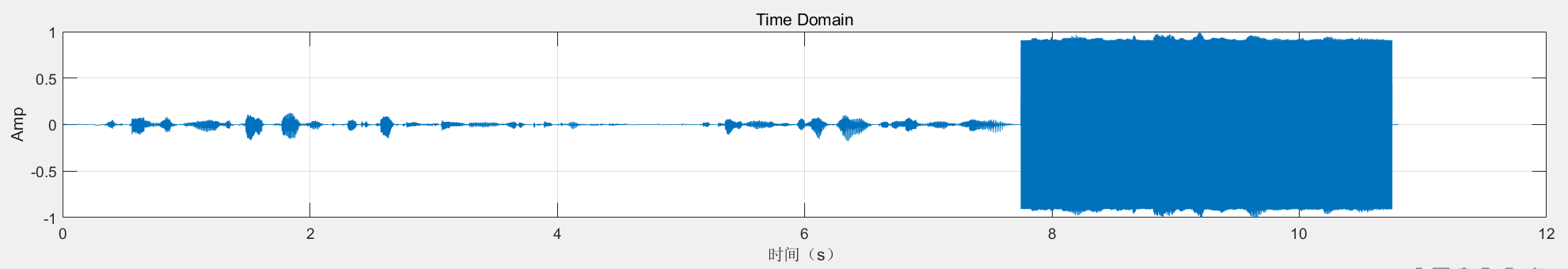#### 频谱分析

使用快速傅里叶变换FFT对时域信号进行频谱分析，绘制的频域图反映出有四段噪声频段，后续消除噪声工作就是对这四个频段进行滤波。

L = 2^nextpow2(L); % 先从原始信号长度确定下一个 2 次幂的新长度,用尾随零填充信号以改善 fft 的性能
Spectrum = fft(audio_data,L); % 快速傅里叶变换，转换为频域
f_x = fs*(0:(L/2))/L; % 定义频域，即变换横坐标量纲为频率
mag_FFT_audio_data = abs(Spectrum / L); % 取幅值，并归一化
ang_FFT_audio_data = angle(Spectrum)*180/pi;

subplot(4, 1, 2);plot(f_x,mag_FFT_audio_data(1 : L/2+1))
title('Frequency Domain');xlabel('频率（Hz）');ylabel('mag');
subplot(4, 1, 3);plot(f_x,ang_FFT_audio_data(1 : L/2+1))
title('Frequency Domain');xlabel('频率（Hz）');ylabel('ang');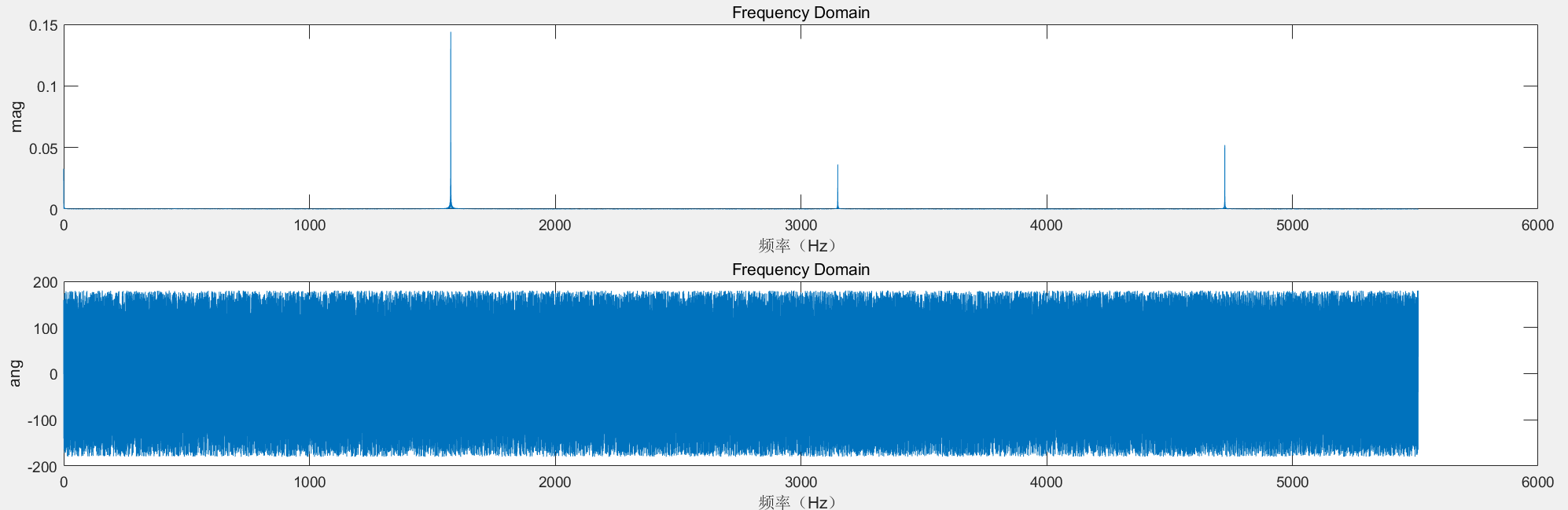#### 绘制声谱图

声谱图能够以颜色反映各频段信号强度随时间的变化和分布情况，如图所示，从第8秒左右开始有4个频段的强度持续较高，这就是上面频域分析出的4个噪声频段。但需要注意到，在噪声的开始和结束时刻，所有频段的强度都很高，在图中显示为两条垂直的黄线，这两条线仅靠滤波无法消除。

subplot(4, 1, 4)
spectrogram(audio_data,hann(256),250,256,fs,'yaxis');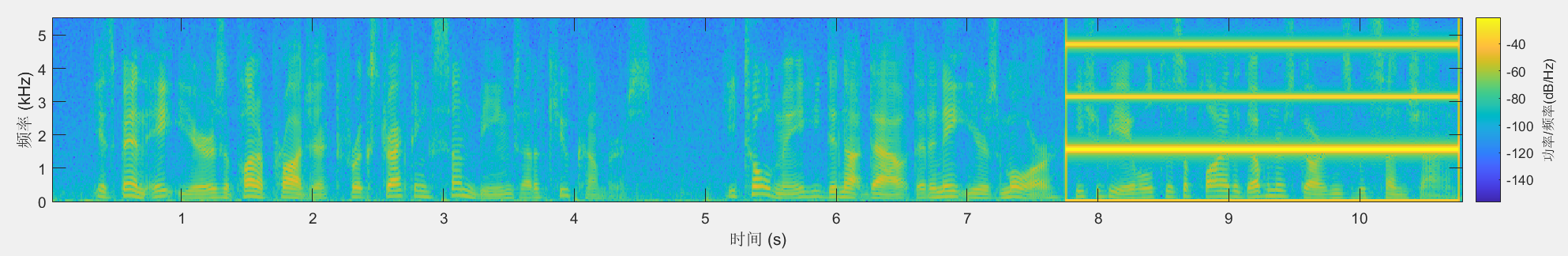### Step2：滤波

#### 滤波法1——手动衰减

手动衰减各个噪声频段的幅度，注意FFT变换后的频谱图为偶对称，所以共有4对（8个）频段，逐一消除。

[m, n] = max(Spectrum)
Spectrum(n-850: n+850) = 0; % 第一个噪声频段

[m, n] = max(Spectrum)
Spectrum(n-850: n+850) = 0; % 第二个噪声频段

[m, n] = max(Spectrum)
Spectrum(n-850: n+850) = 0; % 第三个噪声频段

[m, n] = max(Spectrum)
Spectrum(n-850: n+850) = 0; % 第四个噪声频段

[m, n] = max(Spectrum)
Spectrum(n-850: n+850) = 0; % 第五个噪声频段

[m, n] = max(Spectrum)
Spectrum(n-850: n+850) = 0; % 第六个噪声频段

[m, n] = max(Spectrum)
Spectrum(n: n+850) = 0; % 第七个噪声频段

[m, n] = max(Spectrum)
Spectrum(n-850: n+850) = 0; % 第八个噪声频段

% 逆fft获得处理后的音频
ifft_data = real(ifft(Spectrum, L));
audio_data = ifft_data(1:length(audio_data));

##### 滤波效果

绘制滤波后音频信号的时域图、频域图、声谱图，可看见时域图上噪声被消除，频谱图上4个频段的信号全部被消除，声谱图有四条水平的消磨痕迹。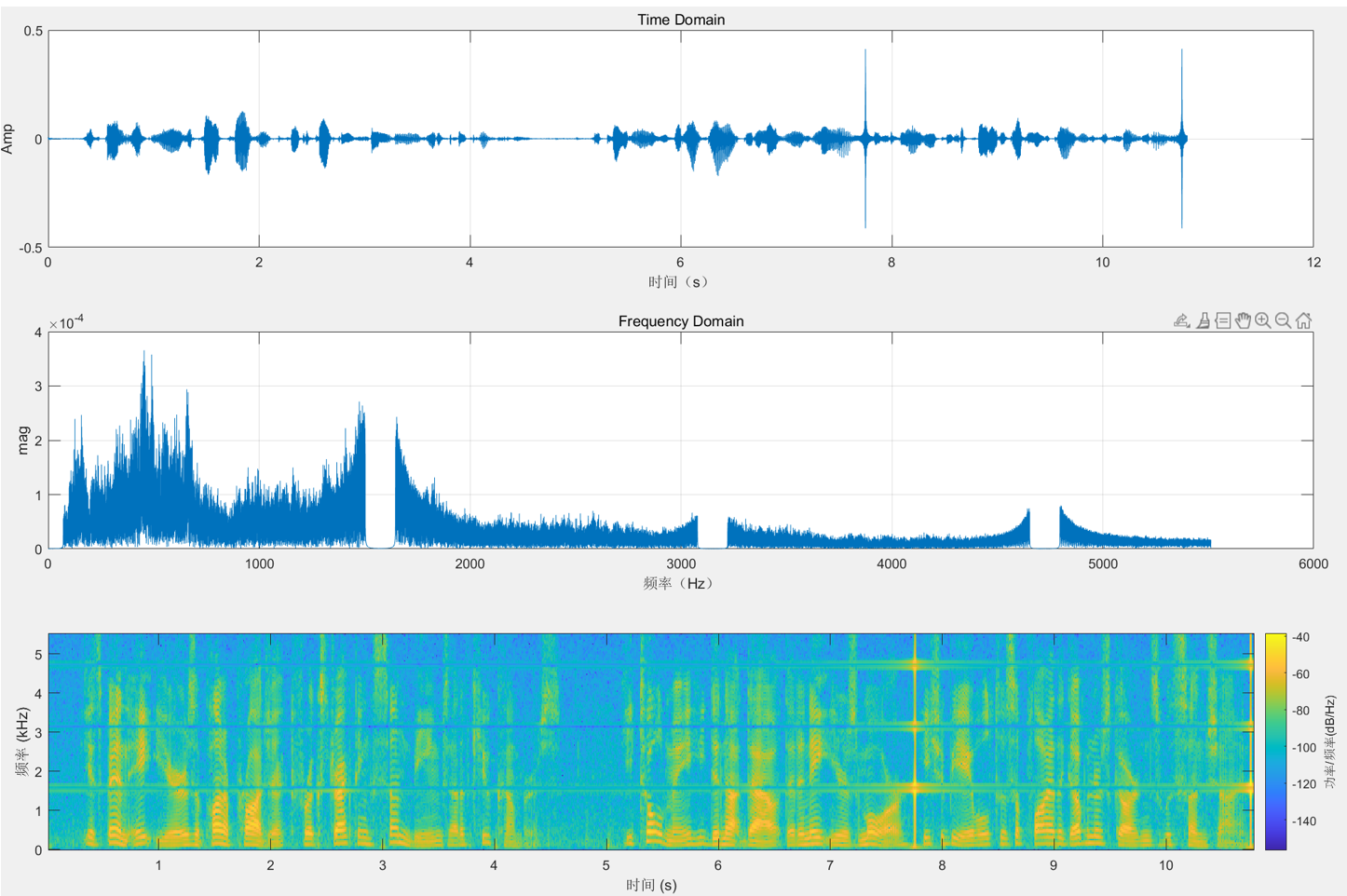#### 滤波法2——数字滤波器

设计梳状滤波器，因为有4个噪声频段，所以设计4个带阻滤波器逐一滤波。

%h1[n] = [1,-2,1];
h1 = [1,-2,1];
h2 = [1,-1.24698,1];
h3 = [1,0.44504,1];
h4 = [1,1.80194,1];
a = [1,0,0];

%滤波过程
audio_data_h1 = filter(h1,a,audio_data);
audio_data_h2 = filter(h2,a,audio_data_h1);
audio_data_h3 = filter(h3,a,audio_data_h2);
audio_data_h4 = filter(h4,a,audio_data_h3);

audio_data = audio_data_h4;

###### 滤波器分析

绘制4个滤波器的幅频、相频、衰减及每次滤波后的信号时域图。### Step3：后续处理

上面已分析，在噪声开始和结束时刻都有全频段的高强度信号，不是滤波能消除的，这里采用时域衰减，即在时域采用同第一种滤波方法相同的思想，手动衰减这两个时刻的信号强度。随后输出处理过的音频文件。

[m, n] = max(audio_data);
audio_data(n-200: n+200) = 0.0001 * audio_data(n-200: n+200); % 衰减第一个高音时段
[m, n] = max(audio_data);
audio_data(n-200: n+200) = 0.0001 * audio_data(n-200: n+200); % 衰减第二个高音频段

% 听一下处理后的音频
% soundsc(audio_data, fs);

% 输出音频文件
audiowrite('SunshineSquare_Processed.wav',audio_data,fs);


使用滤波法2的最终输出信号的分析如下：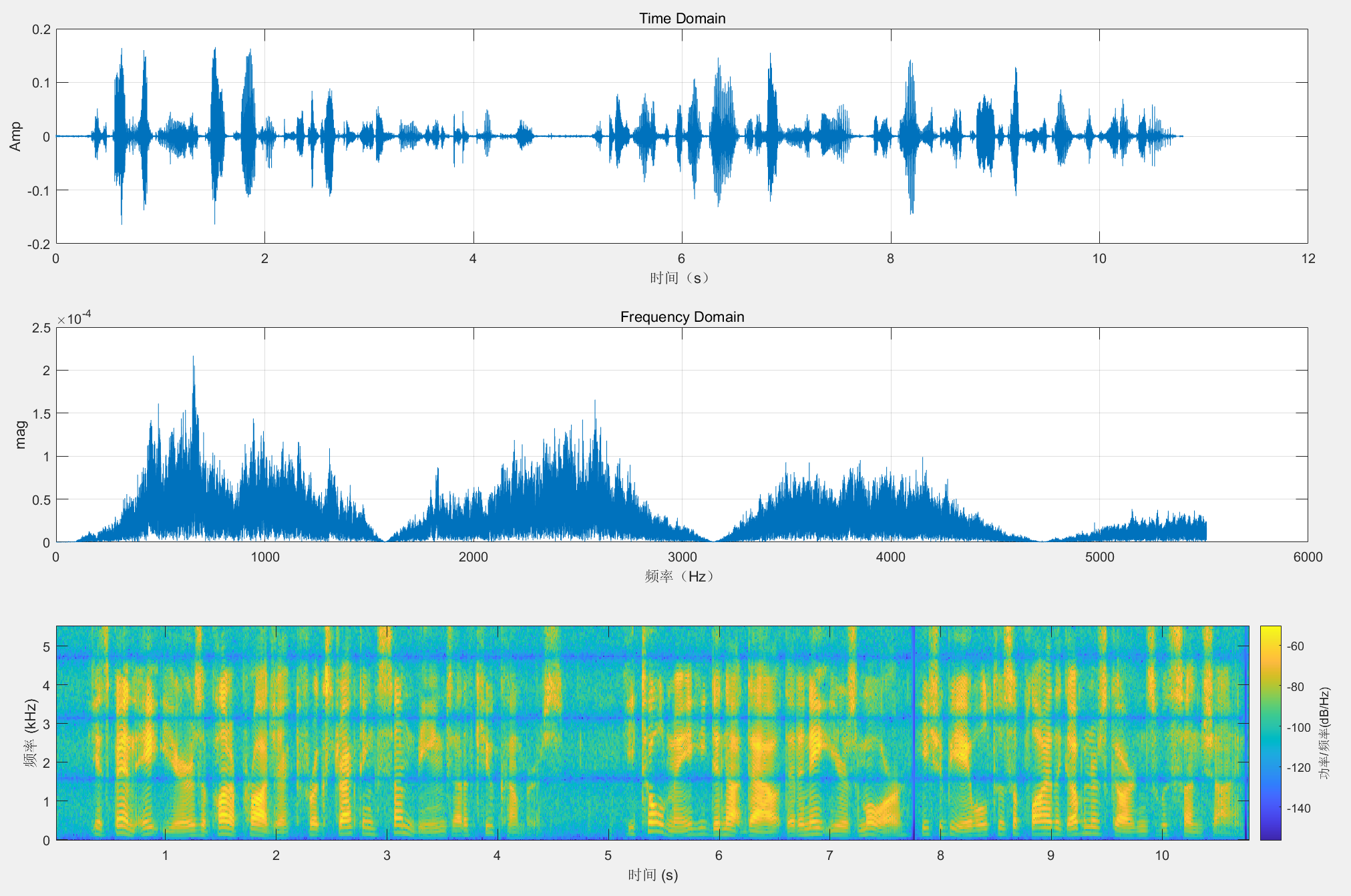展开全文matlab iir滤波器
• 音频信号处理的一些常用matlab代码，大约25个（包含help文件），对初学者有一定帮助
• 复杂的声学环境2.1 声学回声消除2.2 解混响2.3 语音分离2.4 波束形成2.5 噪声抑制2.6幅度控制2.7 前端信号处理的技术路线3. 参考4 .推荐开源项目 原博客地址:https://www.cnblogs.com/LXP-Never/p/13620804.html 1....

### 文章目录

原博客地址: https://www.cnblogs.com/LXP-Never/p/13620804.html

## 1.语音交互

你知道苹果手机有几个麦克风吗？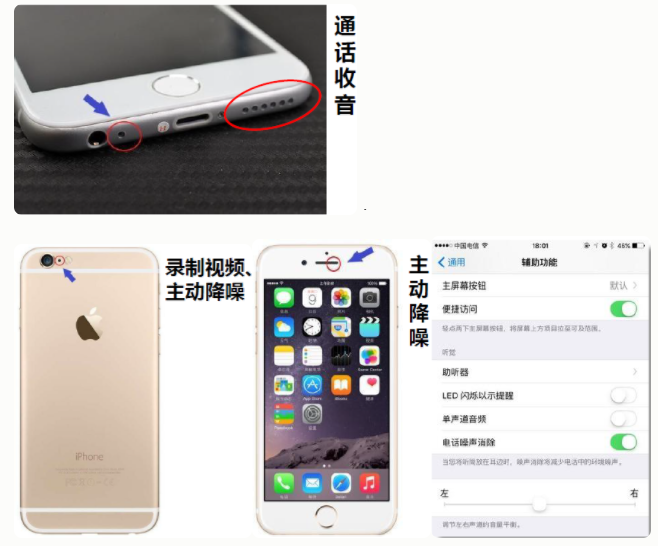语音交互（VUI）是指人与人/设备通过自然语音进行信息传递的过程。

语音交互的优势：

• 输入效率高。语音输入的速度是传统键盘输入方式的3倍以上。例如：语音电视选台、远场语音交互、语音支持组合指令输出（“播放周星驰电影、要免费的、4星以上的”）
• 使用门槛低。人类本就是先有语音再有文字，对于那些无法用文字交互的人来说，语音交互学习成本低，能带来极大的便利。例如：还不会打字的小孩，或者不方便打字的老人家
• 解放双手和双眼，更安全。 例如：车载场景通过语音点播音乐和导航，医疗场景（医生在操作设备的时候，可能还需要记录病例）
传递更多的声学信息。声纹、性别、年龄、情感等。

语音交互的劣势

• 信息接收效率低。 例如：文字能快速阅览概括信息，语音的话必须听完才能理解。
• 复杂的声学环境
• 心理负担。交互方式不一样，例如：不太愿意通过语音来进行交互，特别是在一些公共场合

人机语音交互发展

• 1952年，贝尔实验室，阿拉伯数字识别系统Audrey
• 1962年，IBM-Shoebox
• 2011年，iphone4s，Siri问世
• 2014年，win8，Cortana
• 2014年，Amazon发布echo音箱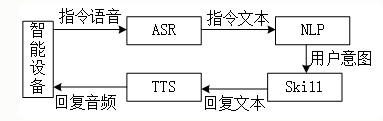应用场景
• 免提通话
• 电话/视频会议
• 手机——Siri、小爱同学
• 车载
• 智能音响——Amazon
• 家居——电视语音点台
总结起来就是：家里、车里、路上

## 2. 复杂的声学环境

现实中的语音交互系统，无一例外的会受到各种环境不利因素的影响，极大影响了交互成功率和用户体验。

• 方向性干扰
• 环境噪声（散射噪声）
• 远讲产生的混响
• 声学回声
痛点：人和机器都听不清

一个成功的语音交互产品，意味着对语音交互的场合和使用模式无约束。

前端语音信号处理的意义：

• 面对噪声、干扰、声学回声、混响等不利因素的影响，运用信号处理、机器学习等手段，提高目标语音的信噪比或主观听觉感受，增强语音交互后续环节的稳健性。
• 让人听清：更高的信噪比，更好的主观听觉感受和可懂度，更低的处理延时。
• 让机器听清：更好的声学模型适配，更高的语音识别性能。

总结：语音信号处理的目标，是为了让人和机器更容易听清语音，让语音交互更加自然和无约束。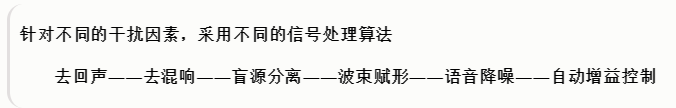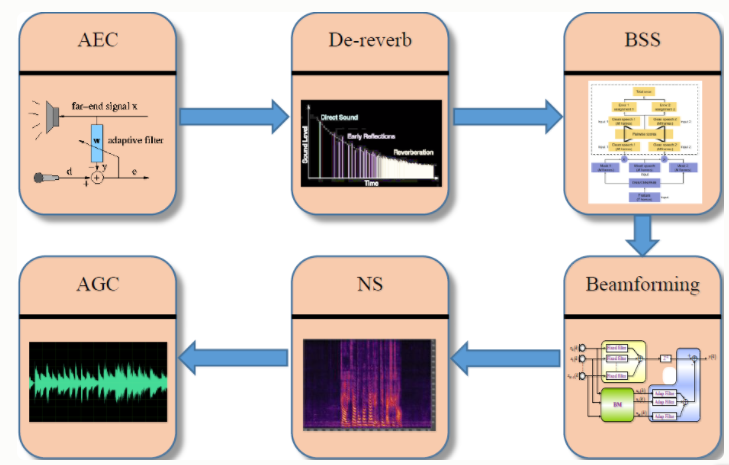### 2.1 声学回声消除

消除设备自身产生的回声干扰，最早应用于全双工语音通信、视频会议，在语音交互中起到打断唤醒的作用

主要模块

• 时延估计（需要把参考信号和输入信号中跟参考信号高度相关的，时间上对齐）
• 线性回声消除（回声消除的核心，设计一组线性自适应滤波器，消除设备自身产生的回声，同时尽可能的保护近端语音不要受到损伤）
• 双讲检测（控制在线性回声消除阶段，究竟什么时刻该做什么事情，有以下几种状态：只有远讲回声信号存在、既有远讲回声信号又有近端的语音信号、
• 残余回声抑制（把前面线性回声消除部分没有消除干净遗漏过来的残余回声，做进一步回声消除）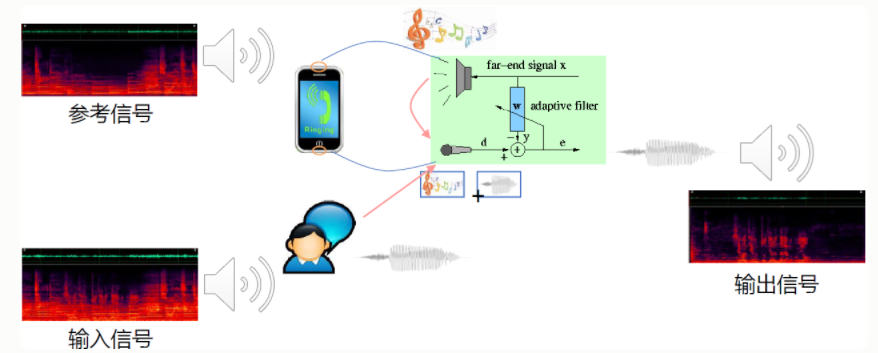• 参考信号：近端扬声器播放的语音信号(没有经过污染的纯净语音)，远端输入信号，近端输出信号
• 输入信号：麦克风接收到的输入信号（参考信号的回声+近端说话语音）
• 输出信号：近端说话语音信号

### 2.2 解混响

混响是由语音的多径效应所产生，在数学表达上是一个近场的纯净语音信号去卷积一个房间的冲击响应函数（RIR），这样的话能得到一个混响的语音信号。我们希望把混响的干扰因素消除掉，技术上有以下几种方法：

• 盲反卷积法[NeelyandAllen，1979]：直接去估计房间冲击响应函数的逆函数，如果把RIR当做是一个滤波器的话，我们直接去估计RIR的一个逆滤波器，然后把逆滤波器作用在带有混响的语音上，就得到了纯净语音信号。（“盲”：即没有任何的先验信息（既不知道原始信号的统计信息，也不知道房间的冲击响应函数），这种情况下想要恢复原始语音信号是非常困难的，所以我们只能假设完全没有噪声的场景，并且假设房间的冲击响应函数RIR是不变的，只有在这种比较严格的假设之下，才能得到相对较好的结果，但是这种假设在我们的实际情况当中是不会得到满足的，所以这种技术缺陷也是比较明显的）
• 加权预测误差[WPE，Takuya，2012]：因为语音信号具有线性预测特性(如果把语音信号当做是一系列采样点信号的话，那么下一个采样点可以用当前时刻以及当前时刻之前的若干采样点的值去预测出下一个时刻采样点的值)，WPE认为混响可以分为早期混响和晚期混响，早期混响对于我们人的听觉感受系统没有负向作用，相反可能还有正面作用；晚期混响相对于房间冲击响应的拖尾的声音。那么加权预测误差则是希望估计一个最优的线性预测滤波器，这个滤波器的作用能够将房间冲击响应函数消除晚期混响的影响，多用于多通道。适用于单通道和多通道场景，多通道效果更好。
麦克风阵列波束形成：混响是多路径反射到达麦克风的，所以入射方向是全向的入射，而语音是方向性的入射，所以可以设计一个波束，拾取手机语音入射方向的语音。这样其他方向的混响就会被抑制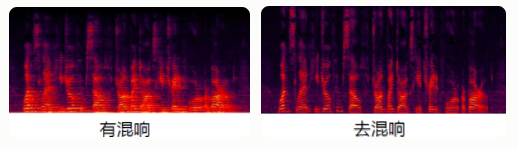### 2.3 语音分离

旨在解决“鸡尾酒会”问题，人类具有这样一种能力，当我们身处多个说话人环境里面，比如：鸡尾酒会，人们可以很容易聚焦我们感兴趣的声音，而当前却没有哪个机器算法能达到人的这种专注注意力的能力，这个问题就引领了语音分离这个方向领域的推进

• 听觉场景分析法[俄亥俄大学 王德亮教授，2004]：将听觉场景分析引入到了计算领域，因此有了Computational Auditory Scene Analysis (CASA)，主要关注的并不仅仅的是语音分离还有语音和其他噪声或音频的分离。技术思路：把鸡尾酒会问题变成一个二分类问题，这个二分类结果称为理想二值掩膜，在时频单元上，噪声占主导，则IBM的值为0；如果目标语音占主导，则IBM的值为1,。这样一来语音分离问题就变成了一个二分类问题。可以通过监督学习来实现，
• 非负矩阵分解[LeeandSeung，2001]：基于统计独立假设，语音信号的稀疏性与谐波特性，把带有干扰的混合语音信号的频谱分解成为一个特征矩阵乘以另一个系数矩阵，那么之后属于不同声源的那些信号的特征就会很自然的聚集到一起，这样一来我们把属于干扰源的特征所对应的系数矩阵进行掩蔽，
基于深度学习的语音分离
• Deep clustering [Hershey, 2016]：通过深度学习的手段，把每一个时频单元结合他的上下文信息把它映射到一个新的空间当中，那么在这个新的空间当中属于同一个说话人的时频单元距离会比较小，可以比较容易的聚类到一起。
• Deep attractor network [Luo and Chen, 2017]：
• Permutation invariant training [Yu, 2017]：解决了置换问题（分离出来的语音信号的顺序）和输出维度问题（不知道有几个声源的情况下）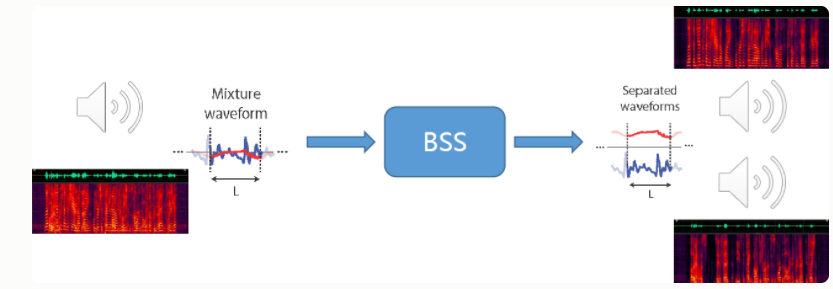### 2.4 波束形成

波束形成技术多用于多通道语音增强、信号分离、去混响、声源定位，
原理：通过空间信息来区分不同位置说话人语音，麦克风阵列形成波束只接受目标说话人语音，其他方向进行屏蔽。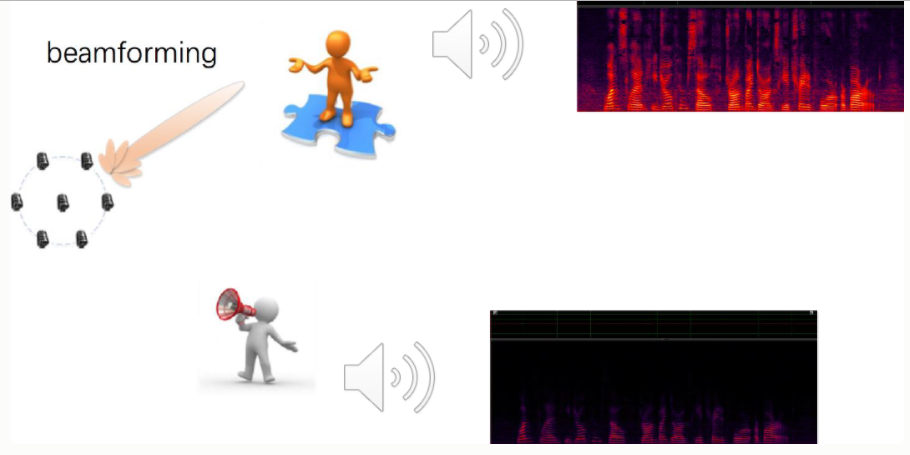主要技术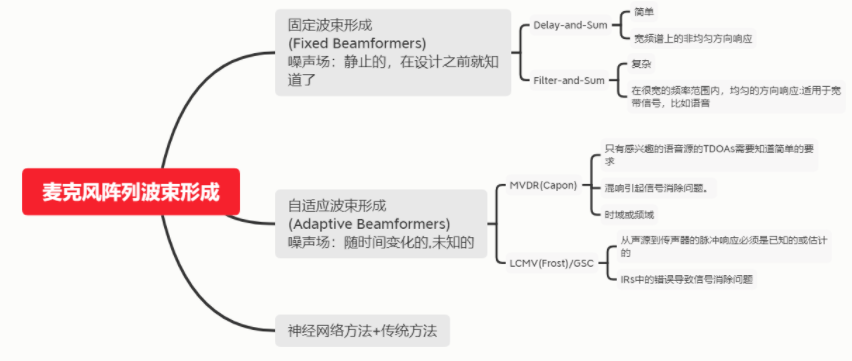### 2.5 噪声抑制

消除或抑制环境噪声，增强语音信号

• 基于统计模型的方法（GMM…）
• 最小均方误差MMSE、最大似然估计ML、最大后验估计MAP
• 基于子空间的方法（MUSIC算法）
• 利用语音和噪声的不相关性，借助特征值/奇异值分解手段分解到子空间处理
• 语音增强的核心在于噪声估计
• 递归平均、最小值追踪、直方图统计是比较常用的噪声估计手段
• 基于深度学习的语音增强方法
• 通过DNN、CNN、RNN或者GAN，在频域或时域实现（多为频域）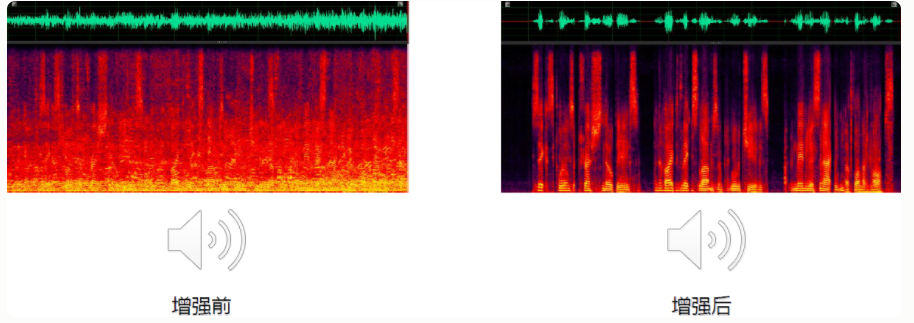### 2.6幅度控制

作用：自动调整信号的动态范围

常用的两种方法

• 动态范围控制（Dynamic Range Control）
• 自动增益控制（Automatic Gain Control）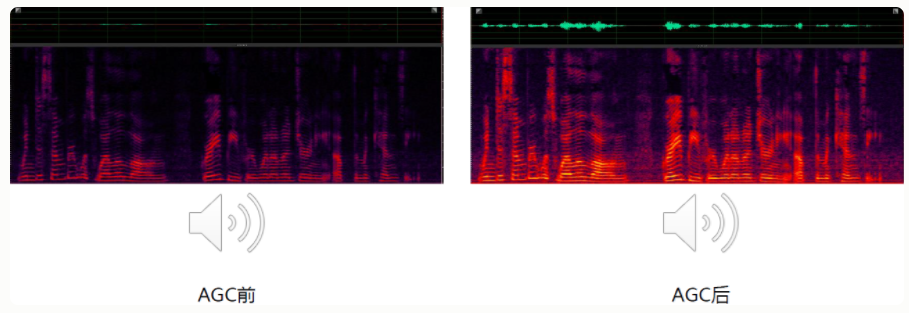### 2.7 前端信号处理的技术路线

传统的前端信号处理方案

处理依据——“规则”

• 客观物理模型，即声音传播的物理规律
• 语音信号的时域、频域和空域特性
针对不同的干扰因素，采用不同的信号处理算法以加以解决

优化目标： 抑制干扰信号，提取目标信号

优化准则： MSE（Mean Square Error）准则

信号处理与深度学习相结合的方案

处理依据——“规则+学习”

• 客观物理模型
• 语音信号的时域、频域、空域特性
• 海量音频数据先验信息

既保留了声音传播的物理规律和信号本身的时域、频域、空域特性，又引入了先验数据统计建模的方法。

优化准则：MSE准则

基于深度学习的前后端联合优化方案

处理依据——“端到端联合建模”

• 输入多通道麦克风信号，输出语音识别结果
• 利用近场数据，仿真得到海量的带有各种干扰的训练数据
• 将前端信号处理与后端ASR声学模型联合建模，用一套深度学习模型完成语音增强和语音识别任务。

优化准则：识别准确率

## 3. 参考

深蓝学院《语音信号处理》课件

奥本海姆，《信号与系统》，电子工业出版社

奥本海姆，《离散时间信号处理》（Discrete Time Signal Processing, Third Edition）

赵力，《语音信号处理》，机械工业出版社

郑君里，《信号与系统》，电子工业出版社，高等教育本科国家级规范教材

韩纪庆，《语音信号处理》，机械工业出版社

张贤达，《现代信号处理》，清华大学出版社

张贤达，《矩阵分析与应用》，清华大学出版社

VanTrees，检测、估计和调制理论（IV)《Optimumarrayprocessing》

## 4 .推荐开源项目

Athena-signal：建议大家下载运行一下，有SEC、NS、波束形成、GSC实际应用的代码

Python for Signal Processing：《Python for Signal Processing: Featuring IPythonNotebooks》对应源码，包含信号处理12大类（采样定理、傅里叶变换、滤波器等）、随机过程15大类（高斯马尔科夫、最大似然等）

VOICEBOX: Speech Processing Toolbox for MATLAB：语音处理工具箱，由MATLAB程序组成。超过100个函数，包含语音增强、ASR等在内。

展开全文语音识别
• 最近在arXiv上看到一篇关于深度学习在音频信号处理中的进展的文章，感觉写的不错，所以根据自己的理解和大家分享一下。如果有些地方博主理解的不对，或者解释不清，可以在下面评论，博主会一一回复。 论文链接：...深度学习 语音识别
• ## 音频信号处理基础知识

千次阅读 热门讨论 2020-10-15 15:41:07
语音信号处理基础知识 1.均值 1.1.均值公式定义 x‾=x1+x2+⋯+xnn=∑j=1nxjn\overline{x} = \frac{x_1 + x_2 + \cdots + x_n}{n} = \frac{\sum^{n}_{j=1}x_j}{n} x=nx1​+x2​+⋯+xn​​=n∑j=1n​xj​​ 1.2.物理...python numpy
• 本论文描述了STM32音频信号分析的设计与实现的经典文档，音频处理方面的朋友可以参考一下。
• labview基于电脑声卡声音信号采集、分析、处理和存储...